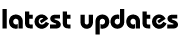# Physics : Waves

A wave is a phenomenon whereby energy is moved without the transference of any material. X-rays, ultraviolet rays, light and radio waves all travel at the same speed through a vacuum. Examples of waves include water waves, sound waves, light and X-rays. There are two main types of waves: transverse and longitudinal. In transverse waves the vibrations are perpendicular to the direction of travel, whereas in longitudinal waves the vibra tions are parallel to the direction of travel.

WAVE LENGTH AND FREQUENCY

The distance between successive wave crests is called the wavelength, X (lamda). The frequency (f) of a wave is defined as the number of complete oscillations per second. Frequency is measured in hertz (Hz). Audible sound frequencies range from 20 Hz (a low rumble) to about 20,000 Hz (a shrill whistle). The speed of a sound wave in air at 20°C (68°F) is 344 m/s, but in water sound travels at 1461 m/s and in steel its speed is 5000 m/s.

PROPERTIES OF WAVES

Waves have several properties, including reflection, refraction, diffraction and interference.

Reflection: Reflection is the process whereby part or all of a wave is returned when it encounters the boundary between two different materials or media. Important example of a wave reflection is an echo, when sound waves bounce off a faraway surface.

Refraction: Refraction is the change of direction of a wavefront as it passes obliquely (at any angle which is not perpendicular or parallel) from one medium to another in which its speed is altered. An example is when light enters a lens or prism – the light is bent. It is the principle of refraction that makes the lenses in spectacles work.

Diffraction : Diffraction occurs when waves passing through a slit which is narrow compared to the wavelength are spread out and depart from the expected straight line direction. This explains how we can hear the words of someone who is facing away from us.

Interference : Interference is the phenomenon that occurs when two or more waves combine together as dictated by the principle of superposition. The superposition principle states that when two waves are in the same place at the same time, their amplitudes (heights) are combined. If  the resultant wave amplitude is greater than that of the individual waves then constructive interference is taking place. If the resultant wave is smaller, then destructive interference is taking place.

ELECTROMAGNETIC WAVES

Electromagnetic waves are caused by a mutual fluctuation in electric and magnetic fields. All the properties of sound and water waves, such as refraction and diffraction, exist in electromagnetic waves, but they differ in that they are able to transmit energy in a vacuum. They travel extremely fast: at 299,792,458 m/s in a vacuum. Electromagnetic waves include light, microwaves,  infrared radiation and X-rays. The electromagnetic spectrum is the collective set of waves over a broad range of wavelengths, from gamma waves (wavelength 10-16 m) to radio waves (wavelength 103 m).

## No comments

Be the first one to leave a comment.My Great Web page